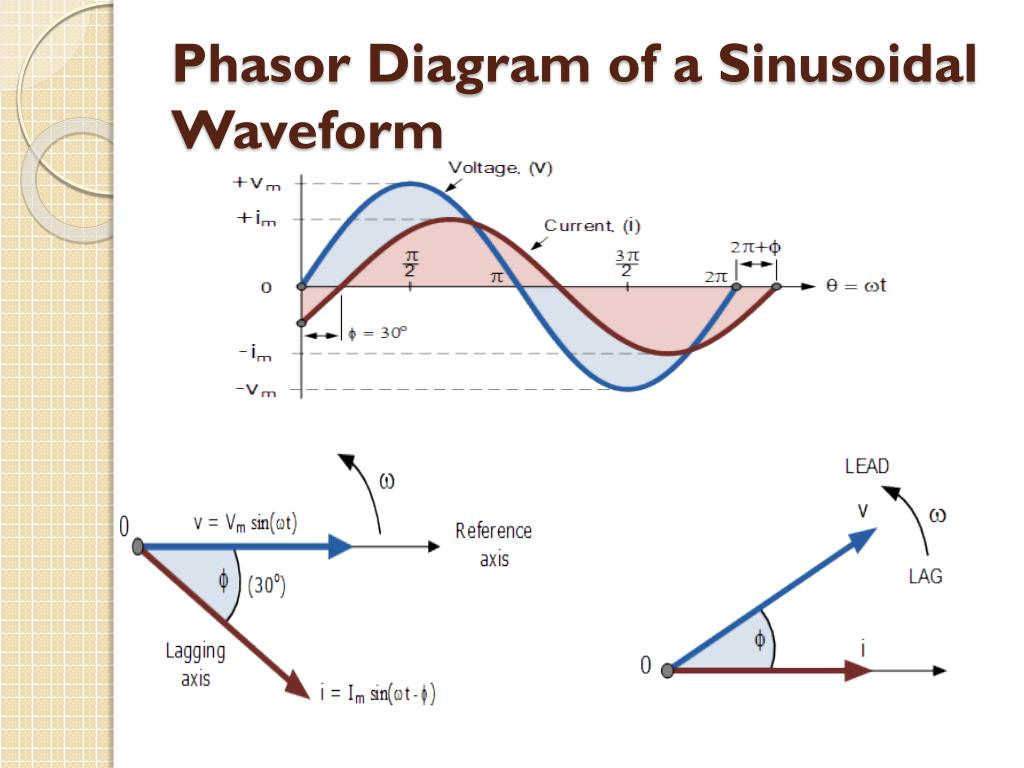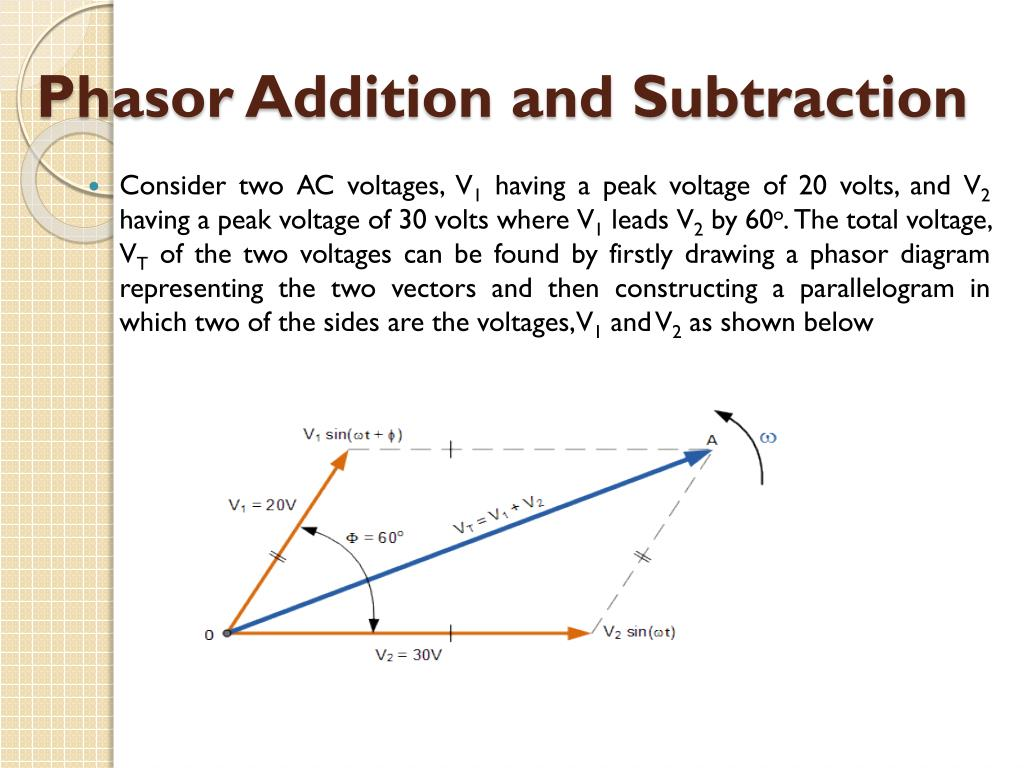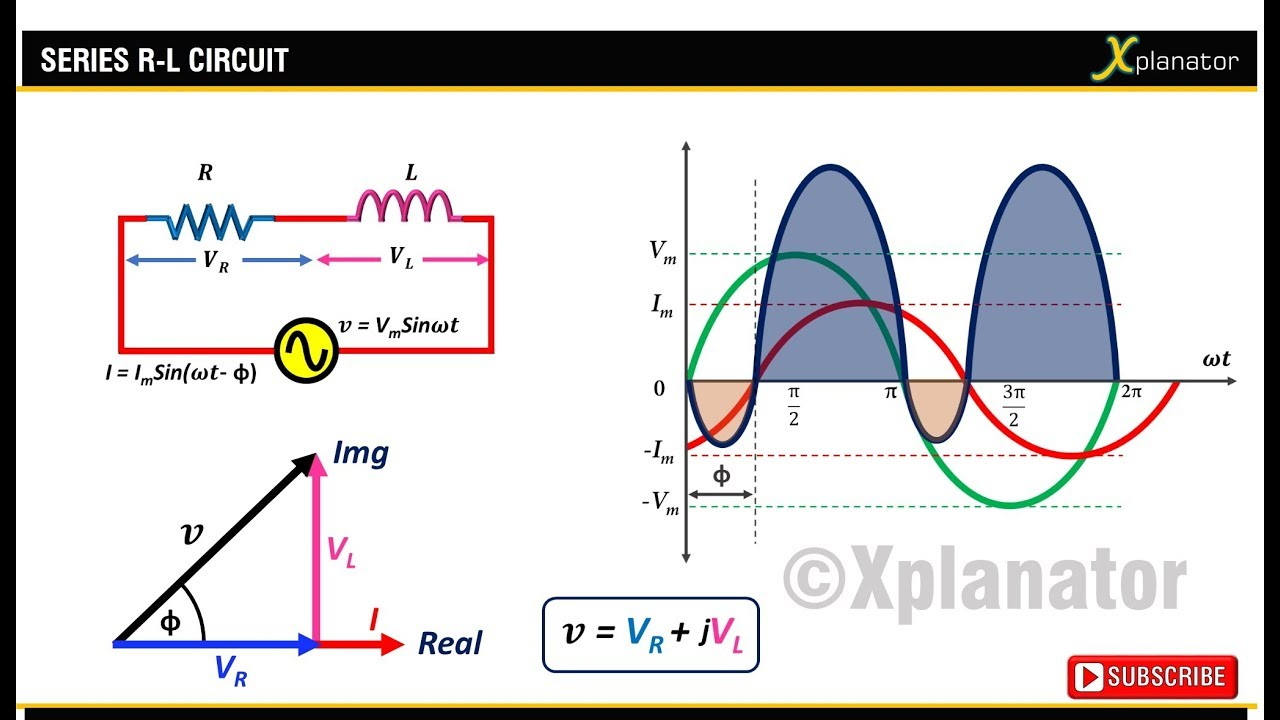# Phasor Representation Of One Phase AC Circuit Presentation

Introduction to Phasor Representation of One Phase AC Circuit
Phasor representation is a mathematical technique used to simplify the analysis of one phase AC circuits.

It allows us to represent time-varying voltages and currents as rotating vectors in a complex plane.

Phasors provide a clear and concise way to analyze AC circuits and calculate their steady-state behavior.
Image Source1

Understanding Phasors
A phasor is a complex number that represents the amplitude and phase of a sinusoidal quantity.

The magnitude of the phasor represents the amplitude of the sinusoidal quantity.

The angle of the phasor represents the phase shift of the sinusoidal quantity.
Image Source2

Phasor Diagrams
Phasor diagrams are graphical representations that show the relationship between different phasors in a circuit.

The length of each phasor represents its magnitude, and the angle represents its phase.

Phasor diagrams help visualize the phasor relationships and simplify complex calculations.
Image Source3

Phasor Arithmetic
Phasor arithmetic involves adding, subtracting, multiplying, and dividing phasors.

When adding or subtracting phasors, we add or subtract their magnitudes and keep the angle of the result.

When multiplying or dividing phasors, we multiply or divide their magnitudes and add or subtract their angles.
Image Source4

Impedance and Phasors
In AC circuits, impedance represents the opposition to the flow of current.

Impedance is also represented as a phasor, combining resistance and reactance.

Phasor representation allows us to easily calculate the total impedance in a circuit.
Image Source5

Applications of Phasor Representation
Phasor representation is widely used in electrical engineering for analyzing power systems, electrical machines, and electronic circuits.

It simplifies calculations and makes it easier to understand the behavior of AC circuits.

Phasor representation is essential in designing and troubleshooting electrical systems. Note: Each slide should also include relevant diagrams, illustrations, or equations to enhance understanding.6